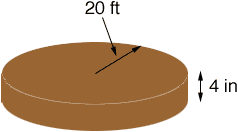Quandaries and Queries Hi,   I need to find out how much sand I would need to order for the base of a horse round pen.  The pen is 40 ft across and I would like a depth of 4 inches.  I have to order the sand in cubic yards.  I am a female senior citizen and I don't have a clue how to figure it.  Please help!  Thanks so much....   Renee Hi Renee, You have a circular slab of sand with a radius of 20 feet and a thickness of 4 inches.The volume of a circular slab isr2t where r is the radius and t is the thickness. Since you want the volume in cubic yards I would first convert all the measurements to yards. There are 3 feet in a yard so 20 feet is 20/3 yards. 4 inches is one-third of a foot so 4 inches is  1/33 = 1/9 yards. Thus the volume is(20/3)21/9 = 15.5 cubic yards. Penny Go to Math Central